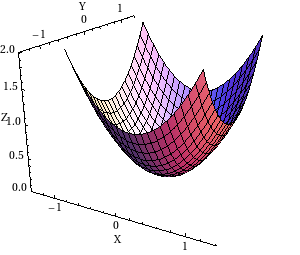# Math Insight

### Image: Elliptic paraboloid on a square domainGraph of the elliptic paraboloid $z=x^2+y^2$ plotted on the square domain $-1 \le x \le 1$, $-1 \le y \le 1$.

Image file: elliptic_paraboloid_square_domain.png

Source image file: elliptic_paraboloid_square_domain.nb
Source image type: Mathematica